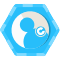## 数据结构之 二叉树的构造与遍历(先序，中序，后序，层次) 原

【推荐】2019 Java 开发者跳槽指南.pdf(吐血整理)>>>``````// 二叉树.cpp : 定义控制台应用程序的入口点。
//

#include "stdafx.h"
#include <iostream>
#define maxSize 10
using namespace std;

typedef struct BinaryTreeNode
{
char data;
BinaryTreeNode * leftChild;
BinaryTreeNode * rightChild;
}Node;
//构造二叉树 使用先序和中序构造一颗二叉树
void MakeBinaryTree(Node** root, char* preOrder, char* midOrder, int length)
{
if (length == 0)
{
(*root) = NULL;
return;
}
(*root) = new Node;
(*root)->data = *preOrder;

char * rootplace = strchr(midOrder, (*root)->data);
if (rootplace == NULL)
{
cout <<"input wrong order sample!"<<endl;
}
int leftTreeLength = strlen(midOrder) - strlen(rootplace);
int rightTreeLength = length - leftTreeLength - 1;

MakeBinaryTree(&(*root)->leftChild, preOrder+1, midOrder, leftTreeLength);
MakeBinaryTree(&(*root)->rightChild, preOrder+leftTreeLength+1, rootplace+1, rightTreeLength);
}

void PostTraverse(Node* root)
{
if (root == NULL)
return;
PostTraverse(root->leftChild);
PostTraverse(root->rightChild);
cout << root->data;
}
void visit(Node *p)
{
printf("%c ",p->data);
}
//先序遍历
void preOrder(Node *p)
{
if(p==NULL)
return;
visit(p);
preOrder(p->leftChild);
preOrder(p->rightChild);
}
//中序遍历
void inOrder(Node *p)
{
if(p==NULL)
return;
inOrder(p->leftChild);
visit(p);
inOrder(p->rightChild);
}
//后序遍历
void postOrder(Node *p)
{
if(p==NULL)
return;
postOrder(p->leftChild);
postOrder(p->rightChild);
visit(p);
}

//层次遍历
typedef struct
{
Node *data[maxSize];
int front;
int rear;
}SqQueue;
void level(Node *&p)
{
Node *q;
SqQueue qu;
qu.front=qu.rear=0;

qu.rear=(qu.rear+1)%maxSize;
qu.data[qu.rear]=p;//进队

while(qu.front!=qu.rear)
{
qu.front=(qu.front+1)%maxSize;
q=qu.data[qu.front]; //出队

visit(q);

if(q->leftChild!=NULL)
{
qu.rear=(qu.rear+1)%maxSize;
qu.data[qu.rear]=q->leftChild;//左孩子进队
}
if(q->rightChild!=NULL)
{
qu.rear=(qu.rear+1)%maxSize;
qu.data[qu.rear]=q->rightChild;//右孩子进队
}
}
}

int _tmain(int argc, _TCHAR* argv[])
{
char pre[] = "abdeijcfg";
char mid[] = "dbiejafcg";	//"bdeijafcg"  "dijebfgca"
Node* r;
MakeBinaryTree(&r, pre, mid, strlen(pre));//构造了一颗二叉树
printf("先序遍历:");
preOrder(r);
printf("\n中序遍历:");
inOrder(r);
printf("\n后序遍历:");
postOrder(r);
printf("\n层次遍历:");
level(r);
return 0;
}``````### 小竹zz2015/01/11
0
0
2137数据结构实验之求二叉树后序遍历和层次遍历

qq_37275680
2017/08/08
0
0

wustor
2017/11/06
0
0
Python 实现二叉树前序，中序，后序，层次遍历

yongxinz
05/16
0
0
LeetCode 二叉树系统题解

11/08
0
0

Java注解合并,注解继承

spring中有时候一个类上面标记很多注解。 实际上Java注解可以进行继承(也就是把多个注解合并成1个) 比如说SpringMVC的注解 @RestController@RequestMapping("/person") 可以合并为一个 @P...

20分钟前
4
0

55分钟前
4
0
Qt编写气体安全管理系统28-模拟工具

4
0

T型人才追梦者

6
0
JVM

4
0